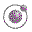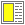Name binding, Recursion, Iteration, and Continuations - slide 13 : 42

Example with cond: american-time
```(define (american-time h m s)
(cond ((< h 0)
(laml-error "Cannot handle this hour:" h))

((and (= h 12) (= m 0) (= s 0))
"noon")

((< h 12)
(string-append
(format-hour-minutes-seconds h m s)
" " "am"))

((= h 12)
(string-append
(format-hour-minutes-seconds h m s)
" " "pm"))

((and (= h 24) (= m 0) (= s 0))
"midnight")

((<= h 24)
(string-append
(format-hour-minutes-seconds (- h 12) m s)
" " "pm"))

(else
(laml-error "Cannot handle this hour:" h))))```The function american-time with helping functions.